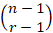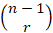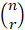-->

# Combination enumeration

Often we are interested in determining the number of groups r different objects that can be formed from a number of n objects. For example, how many groups of 4 different objects can be taken from 5 objects A, B, C, D, and E ?. To answer this, reason as follows. Because there are 5 ways to choose the first object, 4 ways to choose the second object, and 3 ways to select the third object, then there are all 5 x 4 x 3 ways to select group 3 objects if the order of the selected objects is considered. However, because each group of 3 objects, say gurp consisting of objects A, B, and C, will be enumerated 6 times (meaning all ABC permutations, ACB, BAC, BCA, CAB, and CBA will be enumerated if the order of objects- this object is noticed, so the number of groups that can be formed isIn general, because n (n-1) ... (n-r-1) states the number of different ways to select a group of r objects from n available objects if the order is observed and because each group r objects will be counted r! times in this enumeration, the number of groups r different objects that can be formed from a number of n objects is

Sodenotes the number of groups r different objects that can be taken from a number of objects if the order in which they are taken is not observed.

Example Combination Problem 1
A committee of 3 people will be formed from a total of 20 people. How many committees can be formed?
committee that may be formed.

Example Combination Problem 2
From a group of 5 men and 7 women, how many groups of 5 people can be formed consisting of 2 men and 3 women?
how to choose 3 women, then all of them are there

group consisting of 2 men and 3 women.

A useful combinatorial identity is

Equation 1 can be proven by analysis or through the following combinatorial arguments. Suppose we have a group of n objects and focus on any of these objects, name objects number 1. Now there isa combination of size r containing object number 1 (because of this combination this is formed by taking r-1 from the remaining n-1 objects). In addition, there arecombinations of size r that do not contain objects number 1. Because everything is therea combination of size r, then Equation 1 is proven.

is often called a binom coefficient because of its important role in the binomic theorem.

Two proofs for this binom theorem will be given. The first is evidence through mathematical induction, while the second is evidence through combination arguments.

EVIDENCE OF THEORY OF BINOM THROUGH INDUCTION: If n = 1, Equation 2 will be reduced to

Suppose Equation 2 is true for n-1. Furthermore,

By taking i = k + 1 in the first number and i = k in the second number we get,

In this case the Equation before the last equation above is a result of Equation 1. So the above theorem proves by induction

COMBINATORIAL EVIDENCE FOR BINOM THEORIES. Look again,

This product represents the sum of 2n terms, each of which is a product of n factors. In addition, each of these terms has xi or yi as a factor for every i = 1,2, ..., n. For example

Now, how much of this 2n term has k xi and n-k yi as the factor? Because each term consisting of k xi and (nk) yi matches with the selection of a group k element of n values ​​of x1, x2, x3, ..., xm then there isthe fruit of the tribe this is so. So, by taking xi = x and yi = y, i = 1, 2, ..., n, it is obtained,
Example Combination Problem 3

Example of Combination Problem 4
How many subsets can be formed from a set of n elements?

These results can also be obtained in the following ways. To each element in the set